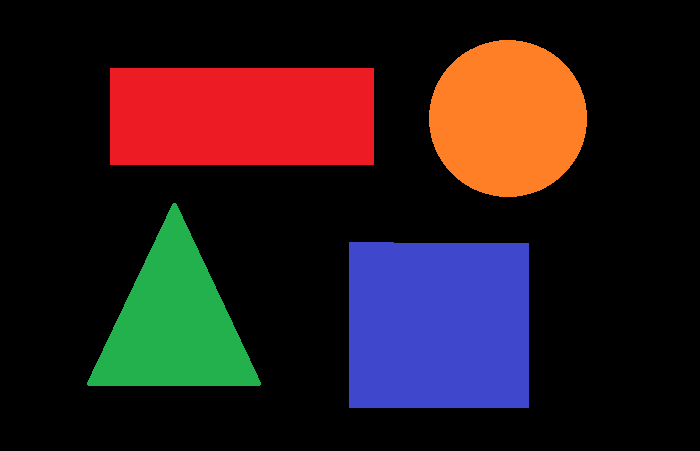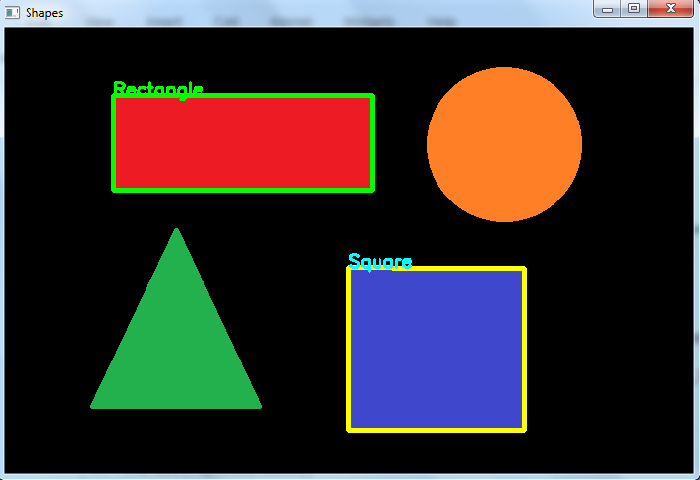# How to detect a rectangle and square in an image using OpenCV Python?

To detect a rectangle and square in an image, we first detect all the contours in the image. Then Loop over all contours. Find the approximate contour for each of the contours. If the number of vertex points in the approximate contour is 4 then we compute the aspect ratio to make a difference between the rectangle and square. If the aspect ratio is between 0.9 and 1.1 we say it is a square else a rectangle See the below pseudocode.

for cnt in contours:
approx = cv2.approxPolyDP(cnt)
if len(approx) == 4:
x, y, w, h = cv2.boundingRect(cnt)
ratio= float(w)/h
if ratio>=0.9 and ratio<=1.1:
cv2.putText('Square')
else:
cv2.putText('Rectangle')


## Steps

You can use the following steps to detect a rectangle and a square in the input image −

Import the required library. In all the following Python examples, the required Python library is OpenCV. Make sure you have already installed it.

import cv2


img = cv2.imread('shapes.jpg')
gray = cv2.cvtColor(img, cv2.COLOR_BGR2GRAY)


Apply thresholding on the grayscale image to create a binary image. Adjust the second parameter to get a better contour detection.

ret,thresh = cv2.threshold(gray,50,255,0)


Find the contours in the image using cv2.findContours() function.

contours, _ = cv2.findContours(thresh, cv2.RETR_TREE, cv2.CHAIN_APPROX_SIMPLE)


Select a contour (say first contour) cnt from the lists of contours. Or loop over all the contours.

Compute the approximate contour points for each contour cnt using cv2.approxPolyDP() function

approx = cv2.approxPolyDP(cnt,epsilon,True)


If the vertex points in the approximate contour approx is 4, then draw the contour on the image.

Compute the aspect ratio of the contour cnt. Set a range of aspect ratios to detect the square. We set it [0.9, 1.1]. If the ratio is between 0.9 and 1.1, the detected contour is a square else it is a rectangle.

Display the image with detected rectangle and square and drawn contours.

cv2.imshow("Shapes", img)
cv2.waitKey(0)
cv2.destroyAllWindows()


Let's look at some examples for clear understanding.

## Example

In the Python code below, we detect the rectangle and square in the input image.

import cv2
import numpy as np

gray = cv2.cvtColor(img, cv2.COLOR_BGR2GRAY)

ret,thresh = cv2.threshold(gray,50,255,0)
contours,hierarchy = cv2.findContours(thresh, 1, 2)
print("Number of contours detected:", len(contours))

for cnt in contours:
x1,y1 = cnt
approx = cv2.approxPolyDP(cnt, 0.01*cv2.arcLength(cnt, True), True)
if len(approx) == 4:
x, y, w, h = cv2.boundingRect(cnt)
ratio = float(w)/h
if ratio >= 0.9 and ratio <= 1.1:
img = cv2.drawContours(img, [cnt], -1, (0,255,255), 3)
cv2.putText(img, 'Square', (x1, y1), cv2.FONT_HERSHEY_SIMPLEX, 0.6, (255, 255, 0), 2)
else:
cv2.putText(img, 'Rectangle', (x1, y1), cv2.FONT_HERSHEY_SIMPLEX, 0.6, (0, 255, 0), 2)
img = cv2.drawContours(img, [cnt], -1, (0,255,0), 3)

cv2.imshow("Shapes", img)
cv2.waitKey(0)
cv2.destroyAllWindows()


Consider the following image as the Input File in the above program code.## Output

When you execute the above code, it will produce the following output.

Number of contours detected: 4


And we get the following window, showing the output −In the above output image, one rectangle and one square are detected.